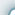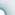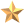Coming Soon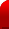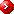New Elem Mathematics 3A (Chp 1-7) - (IX Grade)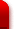Computation and ManipulationEquationsInequalities Indices Evaluation of Algebraic Expressions Algebraic Fractions Equations and Formulae Quadratic Equations Solving Equations by Formula Problems Leading to Quadratic Equation Solving Inequalities Problems Involving Inequalities ApproximationCoordinate Geometry Functions and Variations Graphs Length and Midpoint of a Line Segment Gradient of a Straight Line Equation of a Straight Line Functions Graphs of a Function Inverse Functions Direct and Inverse Variation Gradient of a Graph Solving Simultaneous Equations by Graphs More Problems on GraphsTrigonometry and Bearings Trigonometrical ratios of an Obtuse Angle Cosine Rule Sine Rule Bearings Trigonometrical Problems Involving BearingsNew Elem Mathematics 3B (Chp 8-14) Symmetry Properties of a Circle Angle Properties of a Circle Sets Useful Geometrical Properties Symmetry Properties of a Circle Angle Properties of a Circle More Angle Properties of a Circle The Idea of a Set Intersection and Union of SetsMore About Sets Rate of Change and Area Transformations The Universal Set Using Venn Diagrams to Solve Problems Rate of Change Areas under Graphs Reflection Rotation Translation Enlargement Shear Stretching Combination of Transformation Statistics Presenting Grouped Data Calculation of the Mean Histogram for Grouped Data Cumulative Frequency Percentiles, Quartiles and the Interquartile Range More Problems on Cumulative Frequency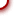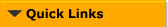cybeRShala Difference Lesson Illustration How It Works Compare Us Request Info Placement Test Tell a friend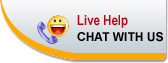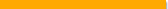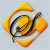Home | FAQ | Tell a Friend | Contact Us | Compare Us | Homeschool Resources | Sitemap cybershala.com Inc. All Rights reserved | Terms of service | Privacy policy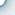s